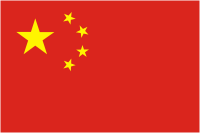China (Hiina) Links to:                      Platepie home  Pictured in Estonia. Countries page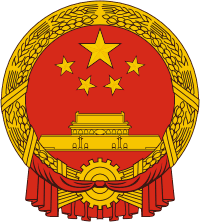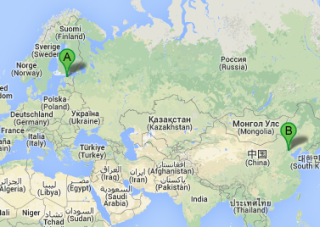Direct distance from Tallinn to Bejing 6382 km screenshot from Google mapsCOMMENTS: normal series since 1994. Plate style since 1994.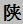= Shaanxi. A = Xi’an. Approximate distance from Tallinn to Xi’an is 7940 km.COMMENTS: normal series since 1994. Plate style since 1994.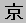= Beijing. N= until 2009 suburbs, since 2009 city area. Approximate distance from Tallinn to Beijing is 8591 km.COMMENTS: normal series since 1994. Plate style since 1994.= Tianjin. A= normal series. Approximate distance from Tallinn to Tianjin is 8615 km.COMMENTS: normal series since 1994. Plate style since 1994.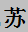=Jiangsu . E= Suzhou. Approximate distance from Tallinn to Suzhou is 9225 km.COMMENTS: normal series since 1994. Plate style since 1994.=Guangdong. B= Shenzhen. Approximate distance from Tallinn to Shenzhen is 9700 km.COMMENTS: normal series since 1994. Plate style since 1994.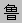=Shandong. B= Qingdao. Approximate distance from Tallinn to Qingdao is 8880 km.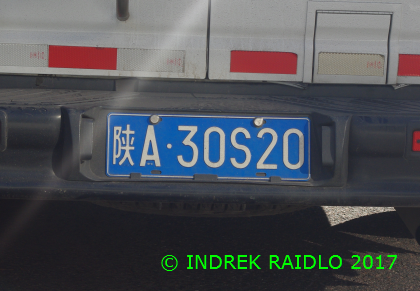COMMENTS: normal series since 1994. Plate style since 1994.= Shaanxi. A = Xi’an. Approximate distance from Tallinn to Xi’an is 7940 km.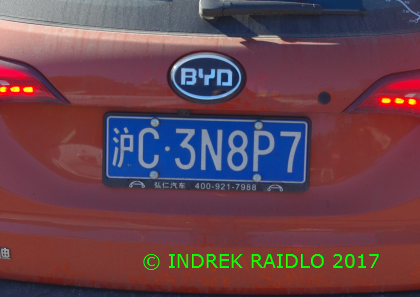COMMENTS: normal series since 1994. Plate style since 1994.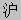=Shanghai. C= Shanghai suburbs. Approximate distance from Tallinn to Shanghai is 9310 km.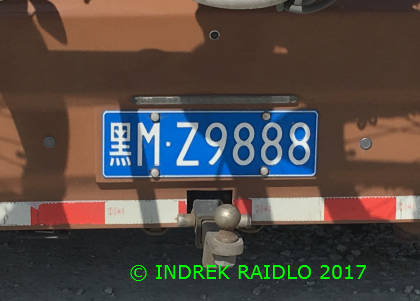COMMENTS: normal series since 1994. Plate style since 1994.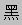=Heilongjiang. M= Suihua. Approximate distance from Tallinn to Suihua is 10139 km.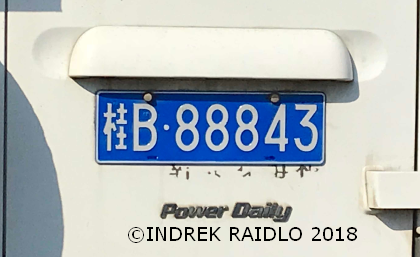COMMENTS: normal series since 1994. Plate style since 1994.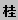= Guangxi Zhuang autonomous region. B= Liuzhou. Approximate distance from Tallinn to Liuzhou is 7408 km.COMMENTS: trailer series since 1994. Plate style since 1994.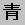=Qinghai. A = Xining.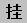= suffix for trailer plates. Approximate distance from Tallinn to Xining is 7155 km.COMMENTS: trailer series since 1994. Plate style since 1994.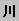=Sichuan. A = Chengdu.= suffix for trailer plates. Approximate distance from Tallinn to Chengdu is 8496 km.COMMENTS: (over 4500 kg) large vehicle series since 1994. Plate style since 1994.=Qinghai. A = Xining. Approximate distance from Tallinn to Xining is 7155 km.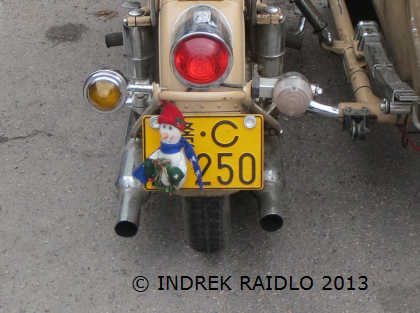COMMENTS: (over 50 cc) motorcycle series since 1994. Plate style since 1994.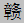=Jiangxi. C = Yichun. Approximate distance from Tallinn to Yichun is 9097 km.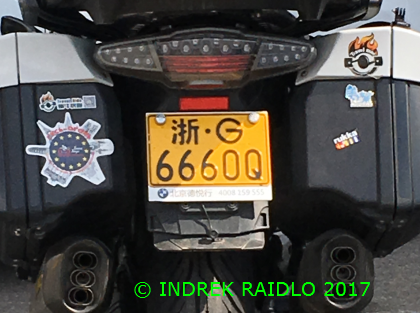COMMENTS: (over 50 cc) motorcycle series since 1994. Plate style since 1994.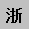=Zhejiang. G = Jinhua. Approximate distance from Tallinn to Jinhua is 9585 km.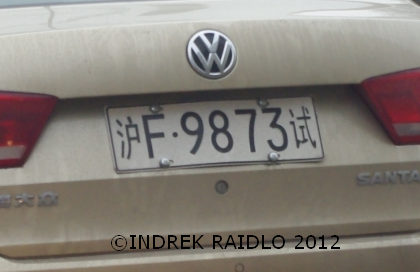COMMENTS: temporary series since 2007.=Shanghai. F= Shanghai city area.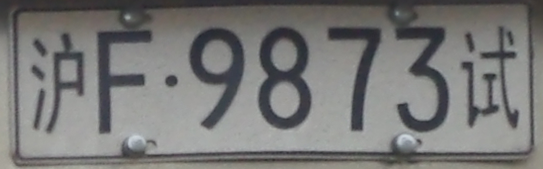Approximate distance from Tallinn to Shanghai is 9310 km.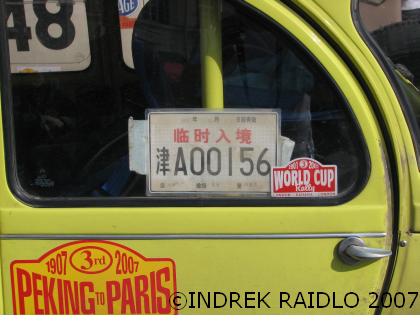COMMENTS: temporary series since 2007. Plate with  ideogram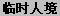for temporary immigrants.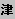= Tianjin. A = Tianjin urban area Approximate distance from Tallinn to Tianjin is 8615 km.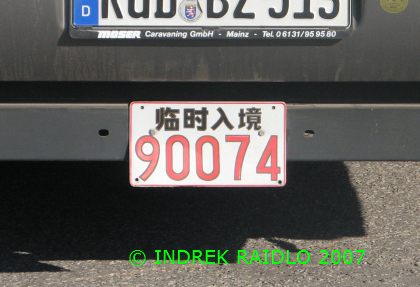COMMENTS: temporary series until 2007. Plate with  Ideogramfor temporary immigrants.

 Previous country: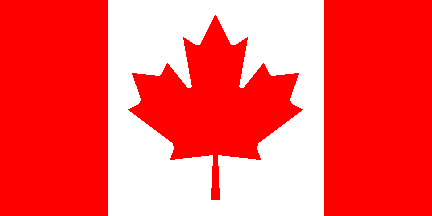SPECIALS: Links to:                               Platepie home  Pictured in Estonia. Countries page Next country: 24.08.2022